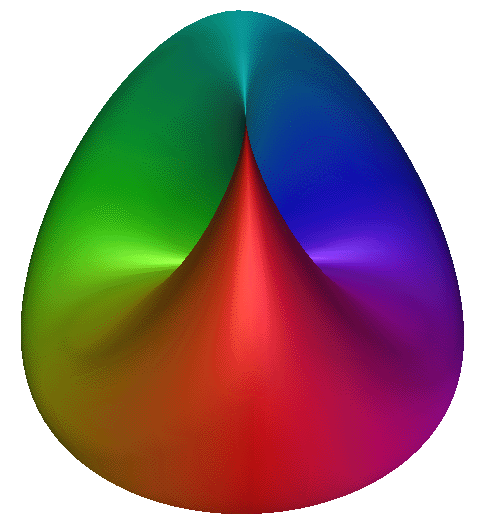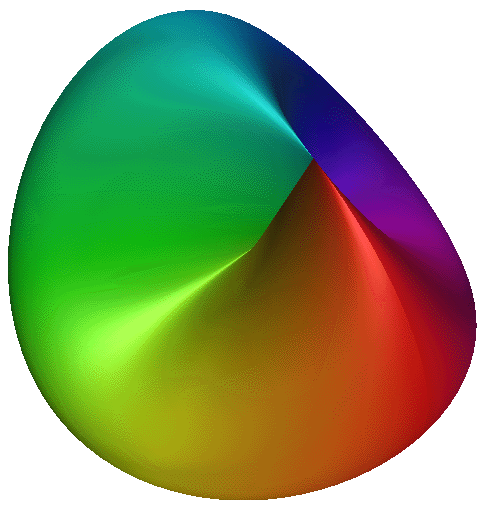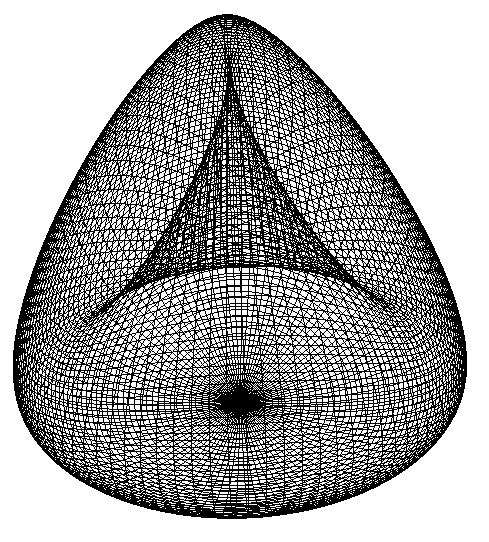# Cross Cap

A self intersecting, single sided surface!

Graphics by Paul Bourke
December 1995

Equations
x = cos(u) * sin(2*v)
y = sin(u) * sin(2*v)
z = cos(v) * cos(v) - cos(u) * cos(u) * sin(v) * sin(v)
0 <= u <= 2and 0 <= v <=/ 2

Implicit surface
4 (x4 + x2 y2 + x2 z2 + x2 z) + y4 + y2 z2 - y2 = 0